# Factoring PolynomialsPage 2

#### WATCH ALL SLIDES

Make a box

Write the factors of the first term.

Write the factors of the last term.

Multiply on the diagonal and add to see if you get the middle term of the trinomial. If so, you’re done!

Slide 11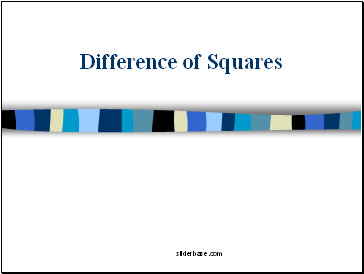## Difference of Squares

Slide 12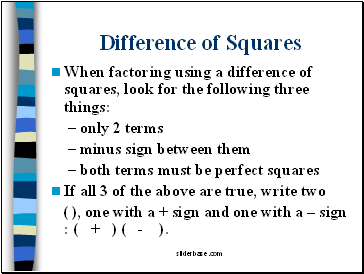Difference of Squares

When factoring using a difference of squares, look for the following three things:

only 2 terms

minus sign between them

both terms must be perfect squares

If all 3 of the above are true, write two

( ), one with a + sign and one with a – sign : ( + ) ( - ).

Slide 13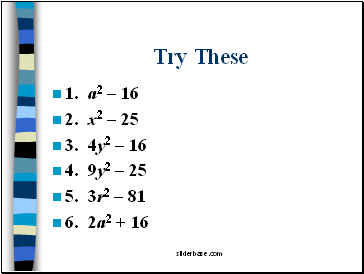Try These

1. a2 – 16

2. x2 – 25

3. 4y2 – 16

4. 9y2 – 25

5. 3r2 – 81

6. 2a2 + 16

Slide 14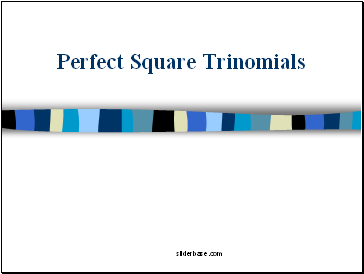## Perfect Square Trinomials

Slide 15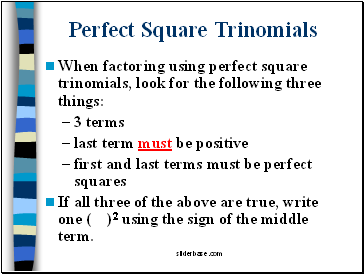Perfect Square Trinomials

When factoring using perfect square trinomials, look for the following three things:

3 terms

last term must be positive

first and last terms must be perfect squares

If all three of the above are true, write one ( )2 using the sign of the middle term.

Slide 16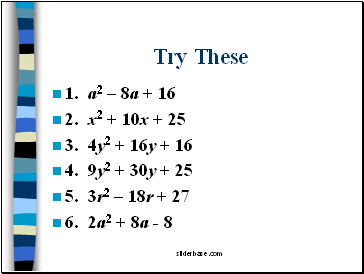Try These

1. a2 – 8a + 16

2. x2 + 10x + 25

3. 4y2 + 16y + 16

4. 9y2 + 30y + 25

5. 3r2 – 18r + 27

6. 2a2 + 8a - 8

Slide 17## Factoring Completely

Slide 18Factoring Completely

Now that we’ve learned all the types of factoring, we need to remember to use them all.

Whenever it says to factor, you must break down the expression into the smallest possible

factors.

Let’s review all the ways to factor.

Slide 19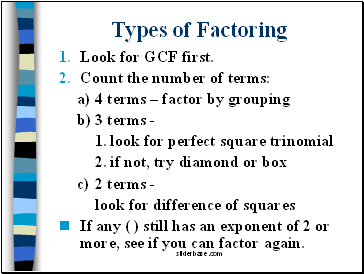Types of Factoring

Look for GCF first.

Count the number of terms:

4 terms – factor by grouping

3 terms -

look for perfect square trinomial

if not, try diamond or box

2 terms -

look for difference of squares

If any ( ) still has an exponent of 2 or more, see if you can factor again.

Slide 20Solving Equations by Factoring

Slide 21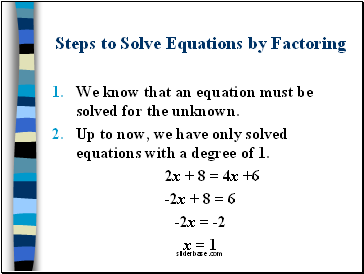## Steps to Solve Equations by Factoring

We know that an equation must be solved for the unknown.

Go to page:
1  2  3